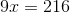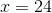Example Questions

Example Question #1 : How To Find The Length Of The Hypotenuse Of A Right Triangle : Pythagorean Theorem

Each of the following answer choices lists the side lengths of a different triangle.  Which of these triangles does not have a right angle?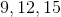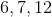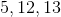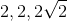Explanation:cannot be the side lengths of a right triangle.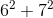does not equal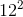. Also, special right triangle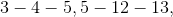and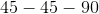rules can eliminate all the other choices.

Example Question #3 : How To Find The Length Of The Side Of A Right Triangle

Given a right triangle with a leg length of 6 and a hypotenuse length of 10, find the length of the other leg, x.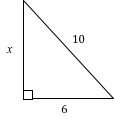16

8

4

64

8

Explanation:

Using Pythagorean Theorem, we can solve for the length of leg x:

x2 + 62 = 102

Now we solve for x:

x2 + 36 = 100

x2 = 100 – 36

x2 = 64

x = 8

Also note that this is proportionally a 3/4/5 right triangle, which is very common. Always look out for a side-to-hypoteneuse ratio of 3/5 or 4/5, or a side-to-side ratio of 3/4, in any right triangle, so that you may solve such triangles rapidly.

Example Question #4 : How To Find The Length Of The Side Of A Right Triangle

In a right triangle a hypotenuse has a length of 8 and leg has a length of 7. What is the length of the third side to the nearest tenth?

2.4
3.6
1.0
3.9
Explanation:

Using the pythagorean theorem, 82=72+x2. Solving for x yields the square root of 15, which is 3.9

Example Question #5 : How To Find The Length Of The Side Of A Right Triangle

Given a right triangle with a leg length of 2 and a hypotenuse length of √8, find the length of the other leg, x.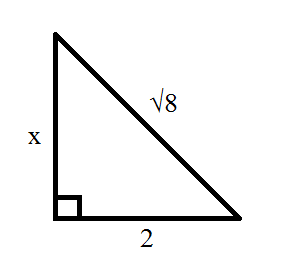4

6

10

2

√8

2

Explanation:

Using Pythagorean Theorem, we can solve for the length of leg x:

x2 + 22 = (√8)2 = 8

Now we solve for x:

x2 + 4 = 8

x2 = 8 – 4

x2 = 4

x = 2

Example Question #6 : How To Find The Length Of The Side Of A Right Triangle

The legs of a right triangle are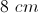and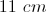. Rounded to the nearest whole number, what is the length of the hypotenuse?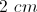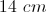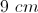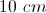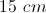Explanation:

Use the Pythagorean Theorem. The sum of both legs squared equals the hypotenuse squared.

Example Question #1 : How To Find The Length Of The Side Of A Right Triangle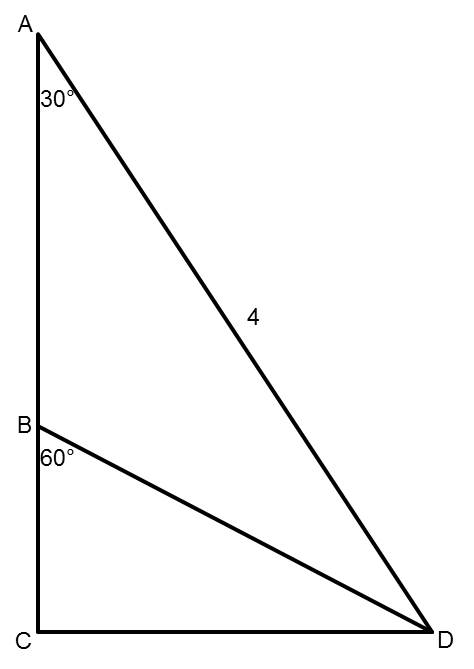Points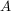,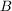, and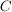are collinear (they lie along the same line).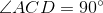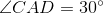,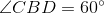,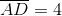Find the length of segment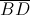.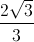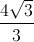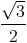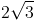Explanation:

The length of segmentisNote that triangles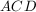and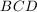are both special, 30-60-90 right triangles. Looking specifically at triangle, because we know that segment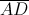has a length of 4, we can determine that the length of segmentis 2 using what we know about special right triangles. Then, looking at trianglenow, we can use the same rules to determine that segmenthas a length of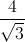which simplifies to.

Example Question #2 : How To Find The Length Of The Side Of A Right Triangle

A handicap ramp is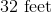long, and a person traveling the length of the ramp goes up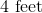vertically. What horizontal distance does the ramp cover?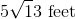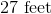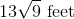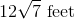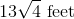Explanation:

In this case, we are already given the length of the hypotenuse of the right triangle, but the Pythagorean formula still helps us. Plug and play, remembering thatmust always be the hypotenuse:State the theorem.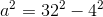Substitute your variables.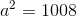Simplify.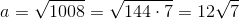Thus, the ramp coversof horizontal distance.

Example Question #1 : How To Find If Right Triangles Are Similar

You have two right triangles that are similar.  The base of the first is 6 and the height is 9.  If the base of the second triangle is 20, what is the height of the second triangle?

30

33

23

25

35

30

Explanation:

Similar triangles are proportional.

Base1 / Height1 = Base2 / Height2

6 / 9 = 20 / Height2

Cross multiply  and solve for Height2

6 / 9 = 20 / Height2

6 * Height2=  20 * 9

Height2=  30

Example Question #2 : How To Find If Right Triangles Are Similar

A right triangle is defined by the points (1, 1), (1, 5), and (4, 1).  The triangle's sides are enlarged by a factor of 3 to form a new triangle.  What is the area of the new triangle?

54 square units

108 square units

None of the answers are correct

36 square units

81 square units

54 square units

Explanation:

The points define a 3-4-5 right triangle.  Its area is A = 1/2bh = ½(3)(4) = 6.  The scale factor (SF) of the new triangle is 3.  The area of the new triangle is given by Anew = (SF)2 x (Aold) =

32 x 6 = 9 x 6 = 54 square units (since the units are not given in the original problem).

NOTE:  For a volume problem:  Vnew = (SF)3 x (Vold).

Example Question #3 : How To Find If Right Triangles Are Similar

On a flat street, a light pole 36 feet tall casts a shadow that is 9 feet long. At the same time of day, a nearby light pole casts a shadow that is 6 feet long. How many feet tall is the second light pole?Explanation:

Start by drawing out the light poles and their shadows.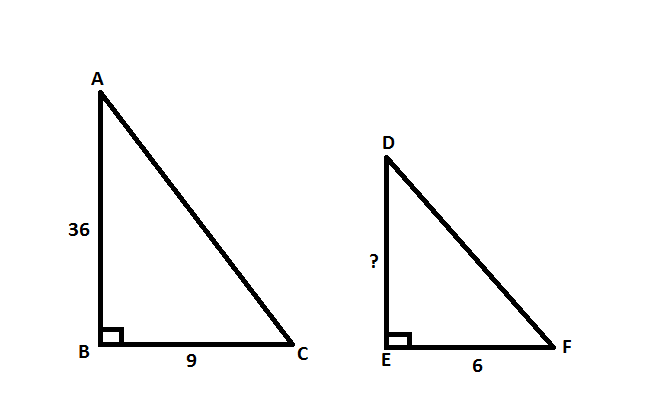In this case, we end up with two similar triangles. We know that these are similar triangles because the question tells us that these poles are on a flat surface, meaning angle B and angle E are both right angles. Then, because the question states that the shadow cast by both poles are at the same time of day, we know that angles C and F are equivalent. As a result, angles A and D must also be equivalent.

Since these are similar triangles, we can set up proportions for the corresponding sides.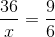Now, solve forby cross-multiplying.